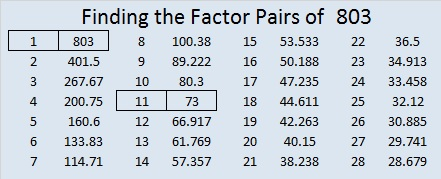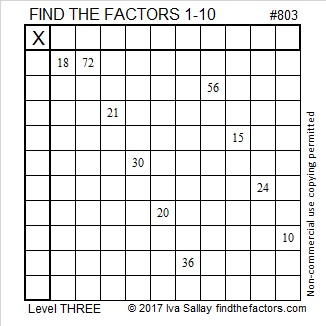# 803 From Top to Bottom

8 – 0 + 3 = 11, so 803 is divisible by 11.

• 803 is a composite number.
• Prime factorization: 803 = 11 x 73
• The exponents in the prime factorization are 1 and 1. Adding one to each and multiplying we get (1 + 1)(1 + 1) = 2 x 2 = 4. Therefore 803 has exactly 4 factors.
• Factors of 803: 1, 11, 73, 803
• Factor pairs: 803 = 1 x 803 or 11 x 73
• 803 has no square factors that allow its square root to be simplified. √803 ≈ 3372546You can solve today’s Level 3 puzzle by starting at the top of the first column, finding the factors of the clues and writing them in the appropriate cells. Then continue to go down that same column, cell by cell, finding factors and writing them down until you reach the bottom. Make sure that both the first column and the top row have each number from 1 to 10 written in them.Print the puzzles or type the solution on this excel file: 10-factors 801-806

Here’s a few more facts about the number 803:

803 is the hypotenuse of a Pythagorean triple:

• 528-605-803 which is 11 times another Pythagorean triple: 48-55-73

803 is the sum of three squares six different ways:

• 27² + 7² + 5² = 803
• 25² + 13² + 3² = 803
• 23² + 15² + 7² = 803
• 21² + 19² + 1² = 803
• 19² + 19² + 9² = 803
• 17² + 17² + 15² = 803

803 is the sum of consecutive prime numbers three different ways. Prime factor 11 is not in any of those ways, but prime factor 73 is in two of them.

• 263 + 269 + 271 = 803, that’s 3 consecutive primes.
• 71 + 73 + 79 + 83 + 89 + 97 + 101 + 103 + 107 = 803, that’s 9 consecutive primes.
• 37 + 41 + 43 + 47 + 53 + 59 + 61 + 67 + 71 + 73 + 79 + 83 + 89 = 803, that’s 13 consecutive primes.

803 is a palindrome in two bases. Why are the numbers similar in these two palindromes?

• 30203 BASE 4 because 3(256) + 0(64) + 2(16) + 0(4) + 3(1) = 803
• 323 BASE 16 because 3(16²) + 2(16) + 3(1) = 803